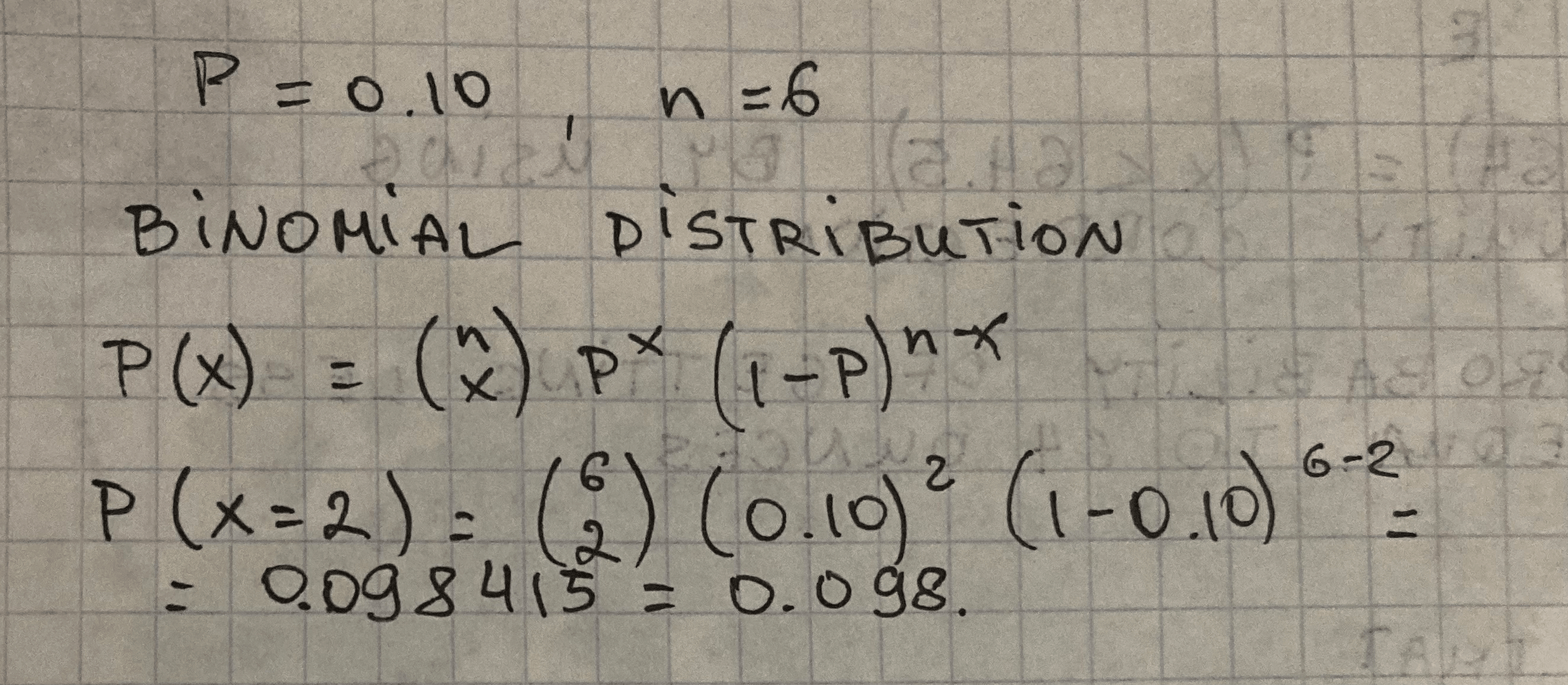#### Didn’t find what you are looking for?

Question# There is a binomial distribution with n = 6 trials, and a probability of success of p = 0.10. Find the probability that the number of successes x is exactly 2. P(x = 2) =…

Binomial probability
ANSWEREDThere is a binomial distribution with n = 6 trials, and a probability of success of p = 0.10. Find the probability that the number of successes x is exactly 2. P(x = 2) =…